## Example Questions

### Example Question #14 : Square Roots And Operations

Simplify: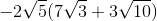Possible Answers: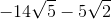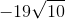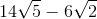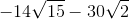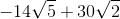Correct answer:Explanation:

To simplify the problem, just distribute the radical to each term in the parentheses.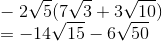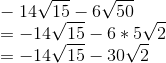### Example Question #15 : Square Roots And Operations

Simplify: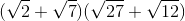Possible Answers: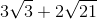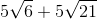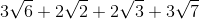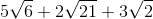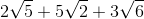Correct answer:Explanation:Let's simplify the right parentheses.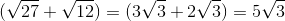Now we can distribute the radical to each term in the parentheses.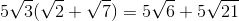### Example Question #16 : Square Roots And Operations

If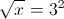what is?

Possible Answers: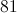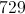Correct answer:Explanation:

Square both sides:

x = (32)2 = 92 = 81

### Example Question #17 : Square Roots And Operations

Simplify in radical form: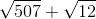Possible Answers: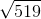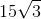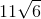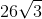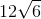Correct answer:Explanation:

To simplify, break down each square root into its component factors: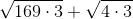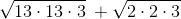You can remove pairs of factors and bring them outside the square root sign. At this point, since each term shares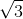, you can add them together to yield the final answer: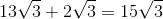### Example Question #36 : Arithmetic

Simplify: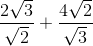Possible Answers: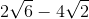None of the other answers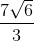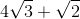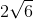Correct answer:Explanation:

Take each fraction separately first:

(2√3)/(√2) = [(2√3)/(√2)] * [(√2)/(√2)] = (2 * √3 * √2)/(√2 * √2) = (2 * √6)/2 = √6

Similarly:

(4√2)/(√3) = [(4√2)/(√3)] * [(√3)/(√3)] = (4√6)/3 = (4/3)√6

Now, add them together:

√6 + (4/3)√6 = (3/3)√6 + (4/3)√6 = (7/3)√6

### Example Question #31 : Basic Squaring / Square Roots

Simplify the following expression: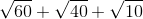Possible Answers: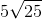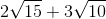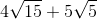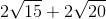Correct answer:Explanation:

Begin by factoring out each of the radicals: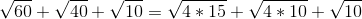For the first two radicals, you can factor out a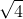or: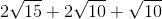The other root values cannot be simply broken down. Now, combine the factors with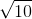: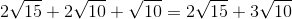This is your simplest form.

### Example Question #1 : How To Add Square Roots

Solve for.

Note,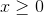: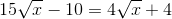Possible Answers: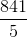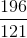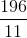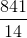Correct answer:Explanation:

Begin by getting yourterms onto the left side of the equation and your numeric values onto the right side of the equation: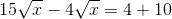Next, you can combine your radicals. You do this merely by subtracting their respective coefficients: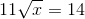Now, square both sides: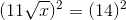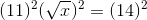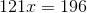Solve by dividing both sides by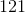: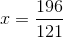### Example Question #179 : Arithmetic

Evaluate: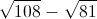Possible Answers: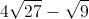None of the available answers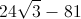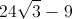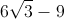Correct answer:Explanation:

Let us factor 108 and 81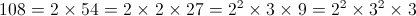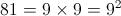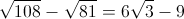### Example Question #1 : Basic Squaring / Square Roots

(√27 + √12) / √3 is equal to

Possible Answers:

5

(6√3)/√3

5/√3

18

√3

Correct answer:

5

Explanation:

√27 is the same as 3√3, while √12 is the same as 2√3.

3√3 + 2√3 = 5√3

(5√3)/(√3) = 5

### Example Question #2 : How To Divide Square Roots

Simplify: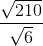Possible Answers: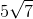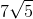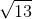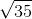Correct answer:Explanation:

When dividing square roots, we divide the numbers inside the radical. Simplify if necessary.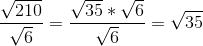### All SAT Math Resources